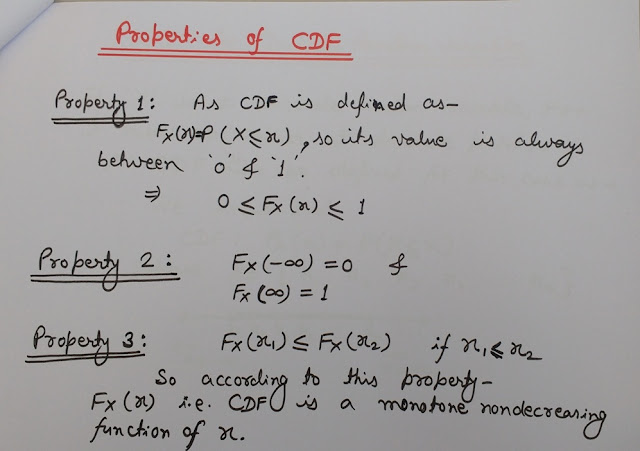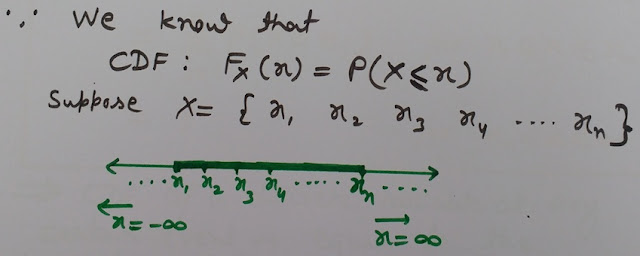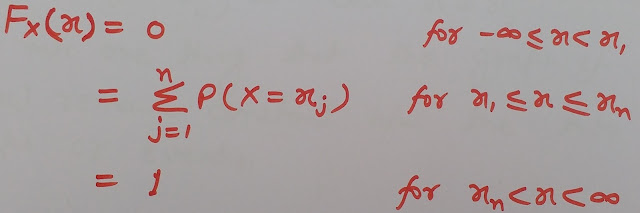## 27 Dec 2018

### Cumulative Distribution Function (CDF) - Properties of CDF - CDF Definition, Basics - Continuous and Discrete CDF

Let's first understand, what is Cumulative Distribution Function (CDF) and it's Definition-

## What is Cumulative Distribution Function (CDF)?

### Definition of CDF-

The Cumulative Distribution Function (CDF) of a random variable 'X' may be defined as the probability that the random variable 'X' takes a value 'Less than or equal to x'.
Mathematically it can be represented as-CDF Formula

Cumulative Distribution Function (CDF) may be defined for-
#Continuous random variables and
#Discrete random variables

Probability Density Function (PDF) - Definition, Basics and Properties of Probability Density Function (PDF) with Derivation and Proof

## Cumulative Distribution Function (CDF) other Names

#Probability distribution function of the random variable
#Distribution function of the random variable
#Cumulative probability distribution function

Following image discusses the 3 Properties of Cumulative Distribution Function (CDF)Properties of CDF (Cumulative Distribution Function Properties)

Joint Probability Density Function (Joint PDF) - Properties of Joint PDF with Derivation- Relation Between Probability and Joint PDF

## Cumulative Distribution Function (CDF) for discrete random variables

If 'X' is a discrete random variable, then it takes on values at discrete points.
Therefore CDF can be defined for this case as shown in the image below-CDF for Discrete Random Variable Explanation

So the CDF for a discrete random variable for the complete range of x can be defined as shown in the image below-CDF for Discrete Random Variable

=> Cumulative Distribution Function (CDF) of a discrete variable at any certain event is equal to the summation of the probabilities of random variable upto that certain event.
As x varies from -∞ to ∞ the graph of CDF i.e. Fx(x) resembles a staircase with upward steps having height P(X=xj) at each x=xj.
But note one thing that the graph of Fx(x) CDF remains constant between the two steps or events.